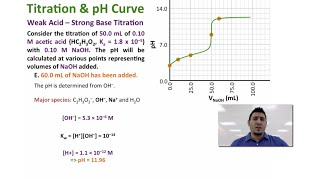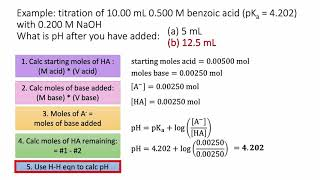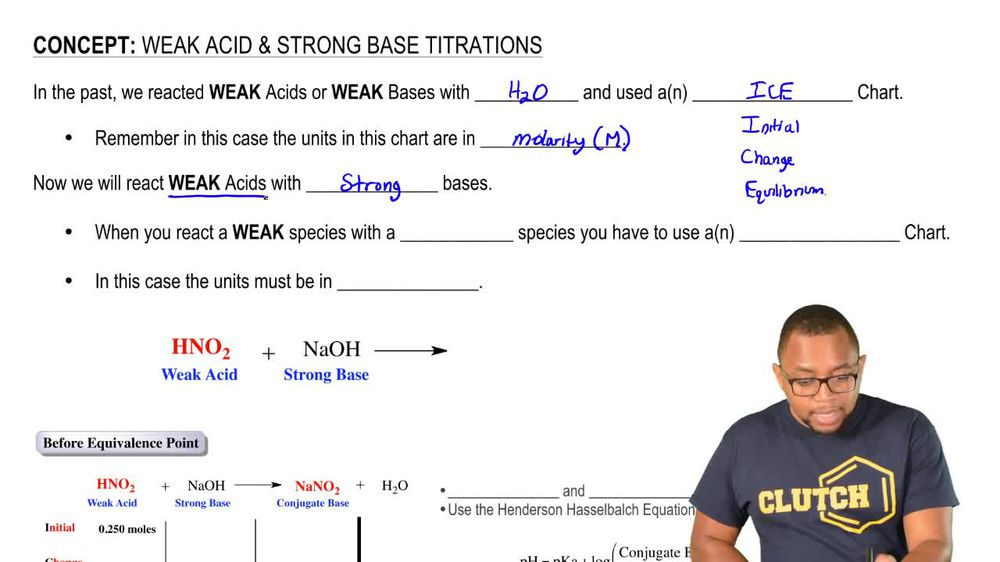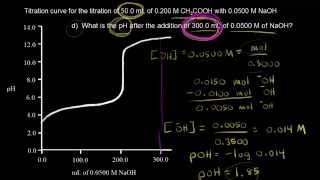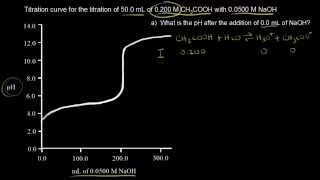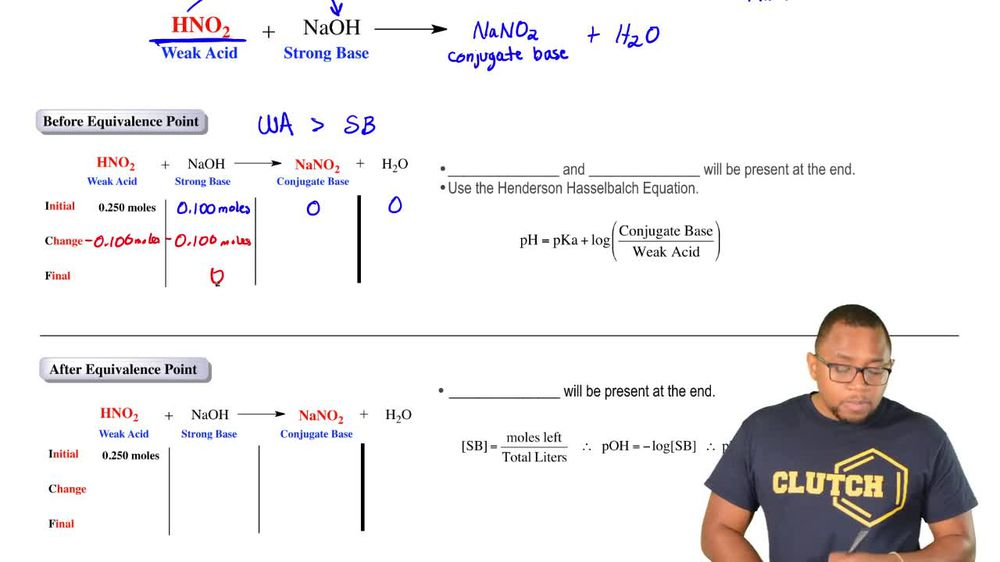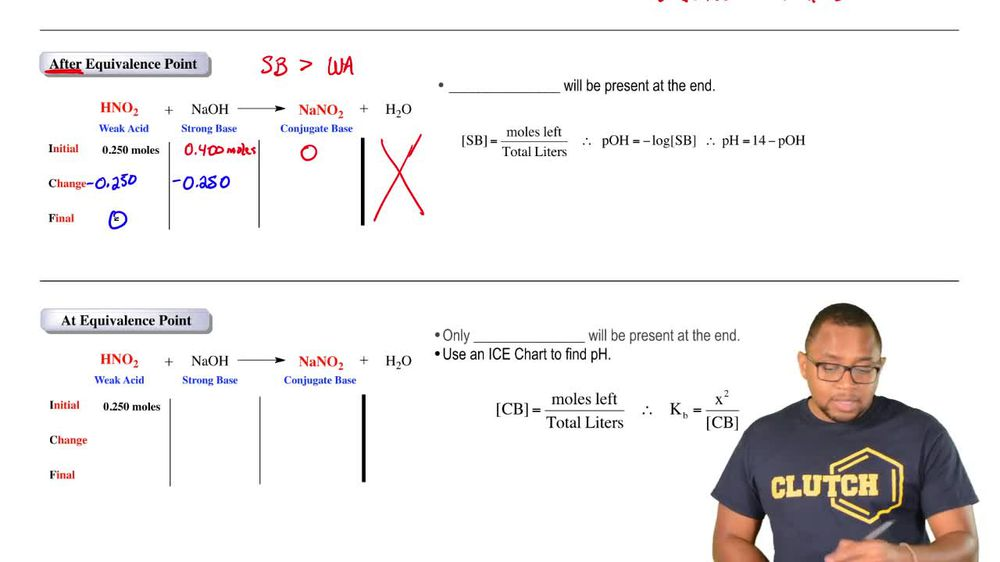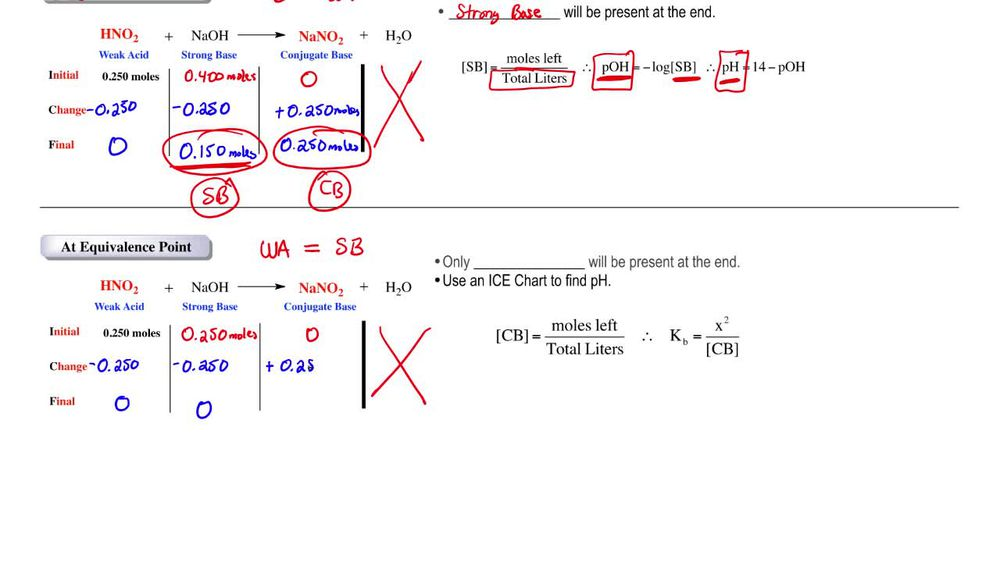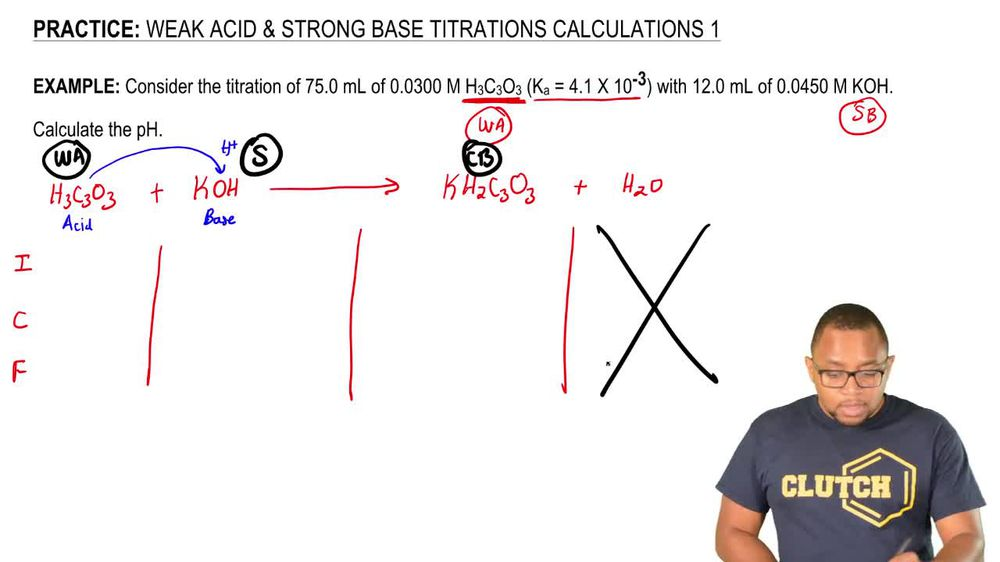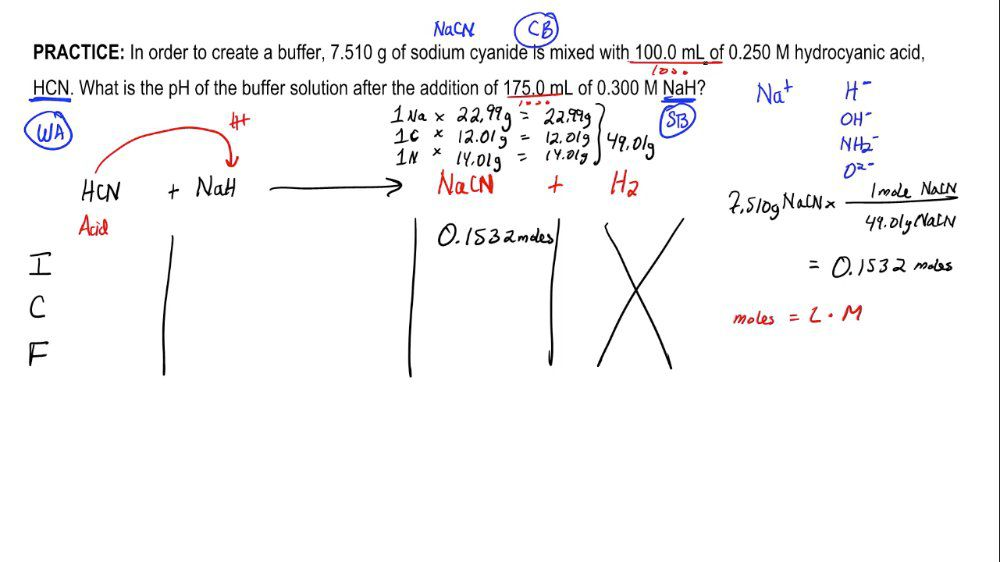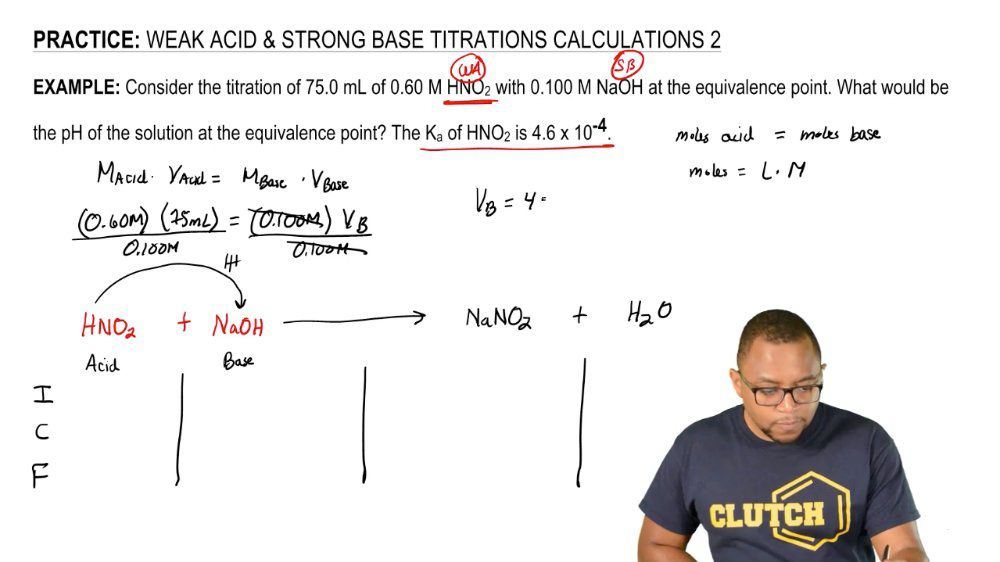Start typing, then use the up and down arrows to select an option from the list.
1. 18. Aqueous Equilibrium2. Weak Acid Strong Base Titrations# Weak Acid-Strong Base Reactions Concept 3

by Jules Bruno
129 views
3
0
So now we're taking a look at calculations after the equivalence point. Now, after the equivalents point, we'd say that are strong species is greater than our weak species. So in this example are strong base will be greater amount than our weak acid. So, for example, we're still starting out with 0.250 moles of my weak acid found. I would say we have 0.400 moles off my strong base here. We'll assume that we're starting out with zero of our conjugate base, but that's not always true. We're just going with zero initially for this example again, just like in a nice try, we ignore water. Now again, Look at the react inside. Look at the react. Inside, the smaller moles will subtract from the larger moles. So negative 0.250 minus 0.250 So at the end, we'll have zero of my weak acid, and we'll still have some strong based remaining 00.150 moles to be in fact, and we're gonna say again, remember the law of conservation of mass matters in the created nor destroyed? We lost 0.250 moles on the reacting side on Lee to gain it back on the product side. Now, At the end of this, what do we have remaining? Well, we have some strong base remaining and we have some contact base remaining. We no longer have a buffer because, remember, a buffer is weak acid and conjugate base. There is no more weak acid, so there is no longer a buffer. Therefore, we cannot use the Henderson Hasselbach equation. What's important here, though, is we have a strong species remaining, so we have strong base. At the end, the strong base will have a greater impact on the pH than a conjugal base. So Onley focus on the strong base. What we would do is we would take the moles left of the strong base and divided by the total leaders used within the question. We would use that information. Help us find the polarity of the strong base because you have a strong base. You could then take the negative log of that concentration to find p o. H. And once you have P o h, remember that pH equals 14 minus P o h. So again, we're at another spot in terms of our titrate shin. And again, you see that we're taking a very different process that we did from before the equivalents point. So just remember, here the strong bases, greater amount in the weak acid, so we'll have some strong base remaining at the end. With that information, we need to determine its polarity toe first, find the P. O. H. And then finally r ph click onto our last video to see what happens when we're at the equivalents point. What approaches need to be taken to find our Ph.